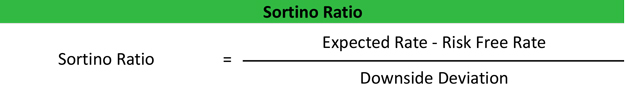# Sortino Ratio

The Sortino ratio is a financial calculation that uses the return below a minimally expectable target to measure a portfolio’s performance adjusted for risk. In other words, it adjusts an investment’s return for risk by looking at potential losses instead of overall volatility to measure the true performance of the investment without the influences of upside volatility.

This measurement is an adaptation of the Sharpe ratio that was developed by William Sharpe to isolate the affects of volatility on investments. Sharpe wanted to mathematically figure out if different investments’ returns went up and down because of investment performance or simply because of market volatility. This concept was a big leap forward in financial mathematics.

The Sortino ratio takes this idea a step further by delineating between general market and harmful volatility. Rather than calculating the affects of the upside and downside of market unrest, this metric only looks at downside volatility by only factoring returns under the minimal acceptable rate that were posted during a time period. Returns that exceed the acceptable rate won’t hurt a portfolio’s score because Sortino doesn’t penalize for volatility like the Sharpe ratio does. This makes sense because we don’t care about volatility if it’s in our favor. We just want to minimize the negative affects of it.

Let’s see how to calculate the Sortino ratio.

## Formula

The Sortino ratio formula is calculated by dividing the difference between the minimally acceptable return and the portfolio’s actual return by the standard deviation of the negative asset returns or the downside deviation.This is kind of a complicated equation, so let’s break down each component.

• <R> = expected return
• Rf = the risk-free rate of return
• od = standard deviation of negative asset returns

Here’s what it looks like in plain EnglishBy removing the minimally acceptable rate of return, also called the risk-free rate, from the expected return, we can see how much the expectations exceed the minimum rate. Then we can adjust it for the negative returns.

## Analysis

Individuals use this calculation to measure the return needed to meet a specific financial goal in the future. For example, an they might use this to figure out how long it will take them to save up a down payment on a car or home.

Investors typically use this figure to measure the risk and performance of investments in a portfolio like mutual funds. SR is also used as a measuring stick for investment managers because it represents the excess returns above the investors’ minimum acceptable rate that the manager was able to achieve for the period.

A higher ratio is always preferred to a lower one because it indicates that the portfolio or investment is operating efficiently and carries a low risk of a large sudden loss. In this way, it does a better job evaluating the overall risk on an investment than the Sharpe method.

The choice to use the Sortino or Sharpe methods to evaluate an investment is solely based on whether you want to analyze the total volatility using the standard deviation or the downside volatility using the downside deviation. Both are commonly used in the investment community for different applications.

Contents